5-68.

### Missing problem for Book int1s => Chapter Ch5 => Lesson 5.2.2 => Problem 5-68

Created from orphaned homework help problem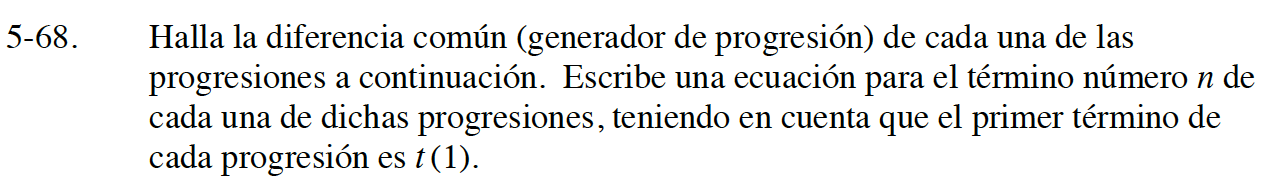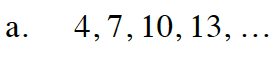m = 3

7 − 4 = 10 − 7 = 13 − 10 = 3

t(n) = 3n + t(0)
t(1) = 3(1) + t(0) = 4
3 + t(0) = 4
t(0) = 1

t(n) = 3n + 1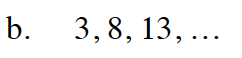m = 5

t(n) = 5n − 2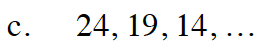t(n) = −5n + 29

m = −5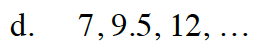9.5 − 7 = 12 − 9.5 = 2.5

m = 2.5

t(0) = 4.5

t(n) = 2.5n + 4.5